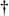FFT INPUT/OUTPUT DATA INDEX BIT REVERSAL

FFT INPUT OUTPUT DATA INDEX BIT REVERSAL

OK, let's look into some of the special properties of the FFT that are important to FFT software developers and FFT hardware designers. Notice that Figure 4-5 was titled "Full decimation-in-time FFT implementation of an 8-point DFT." The decimation-in-time phrase refers to how we broke the DFT input samples into odd and even parts in the derivation of Eqs. (4-20), (4-23), and (4-24). This time decimation leads to the scrambled order of the input data's index n in Figure 4-5. The pattern of this shuffled order can be understood with the help of Table 4-1. The shuffling of the input data is known as bit reversal because the scrambled order of the input data index can be obtained by reversing the bits of the binary representation of the normal input data index order. Sounds confusing, but it's really not—Table 4-1 illustrates the input index bit reversal for our 8-point FFT example. Notice the normal index order in the left column of Table 4-1 and the scrambled order in the right column that corresponds to the final decimated input index order in Figure 4-5. We've transposed the original binary bits representing the normal index order by reversing their positions. The most significant bit becomes the least significant bit and the least significant bit becomes the most significant bit, the next to the most significant bit becomes the next to the least significant bit, and the next to the least significant bit becomes the next to the most significant bit, and so on.[]

[] Many that are first shall be last; and the last first. [Mark 10:31]

Table 4-1. Input Index Bit Reversal for an 8-point FFT

Normal order of index n

Binary bits of index n

Reversed bits of index n

Bit-reversed of order index n

0

000

000

0

1

001

100

4

2

010

010

2

3

011

110

6

4

100

001

1

5

101

101

5

6

110

011

3

7

111

111

7Amazon